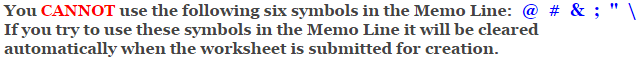# Algebraic Expressions Worksheets

## Evaluating Two Variables Worksheets

This Evaluating Two Variables Worksheet will create algebraic statements
for the student to evaluate.

You may select from 2, 3 and 4 terms with addition, subtraction, multiplication, and division.

### Type of Problems

 2 Terms (Addition and Subtraction) 3 Terms (Addition and Subtraction) 4 Terms (Addition and Subtraction) 3 Terms (Multiplication, Addition, and Subtraction) 4 Terms (Multiplication, Addition, and Subtraction) 2 Terms (Division, Addition, and Subtraction) 3 Terms (Division, Addition, and Subtraction)

### Type of Numbers to Use for the Variables

 Whole Numbers Integers

### Language for the Algebraic Expressions Worksheet

 English German Albanian Spanish Swedish Italian French Turkish Polish Norwegian

### Memo Line for the Algebraic Expressions Worksheet

You may enter a message or special instruction that will appear on the bottom left corner of the Algebraic Expressions Worksheet.### Algebraic Expressions Worksheet Answer Page

Now you are ready to create your Algebraic Expressions Worksheet by pressing the Create Button.

If You Experience Display Problems with Your Math Worksheet